Ex 4.2

Chapter 4 Class 7 Simple Equations
Serial order wise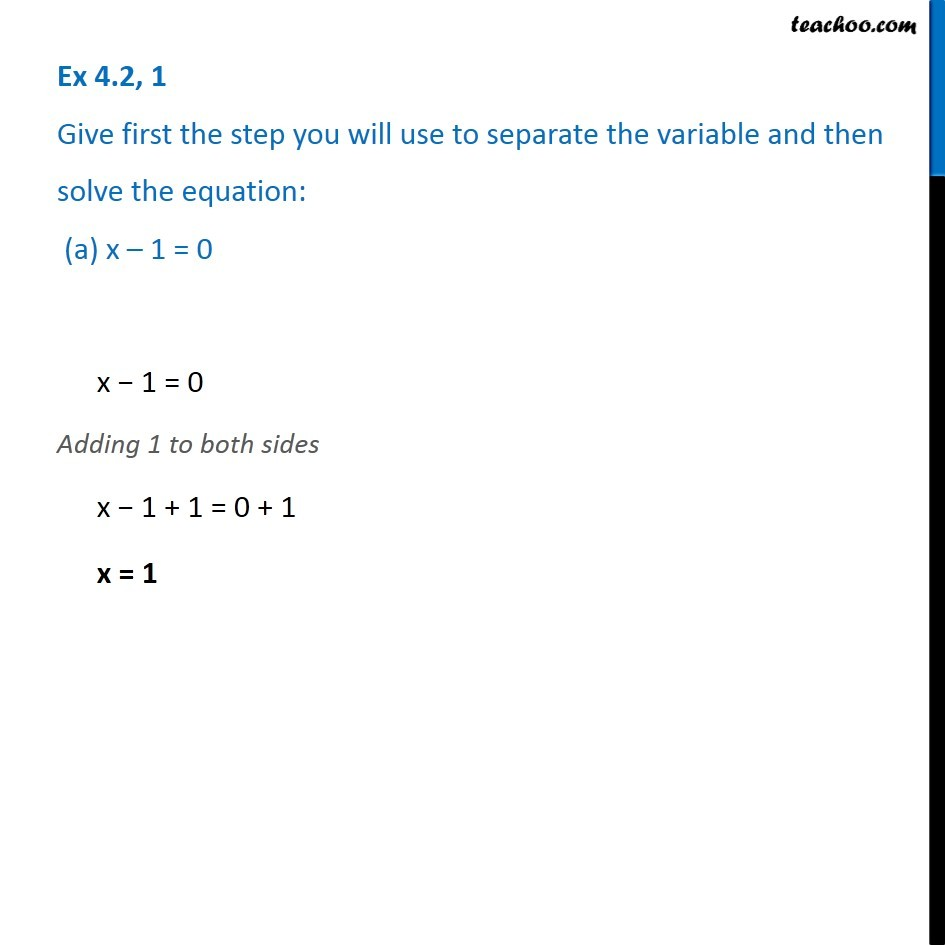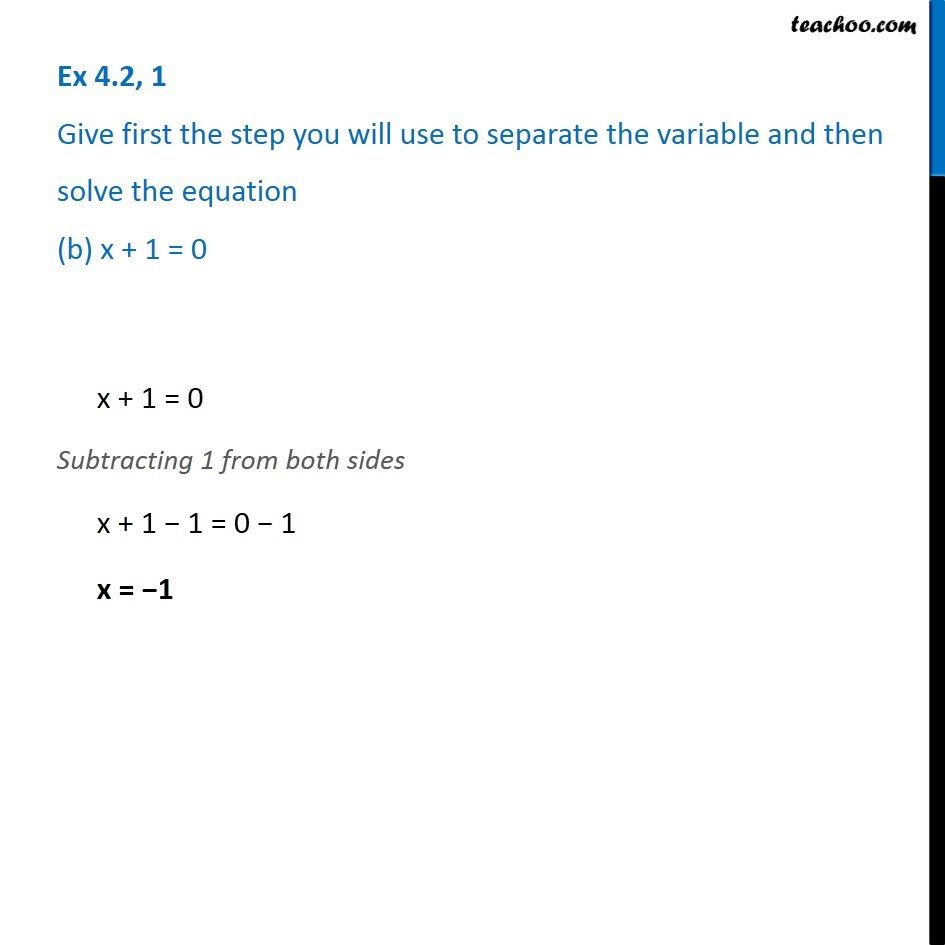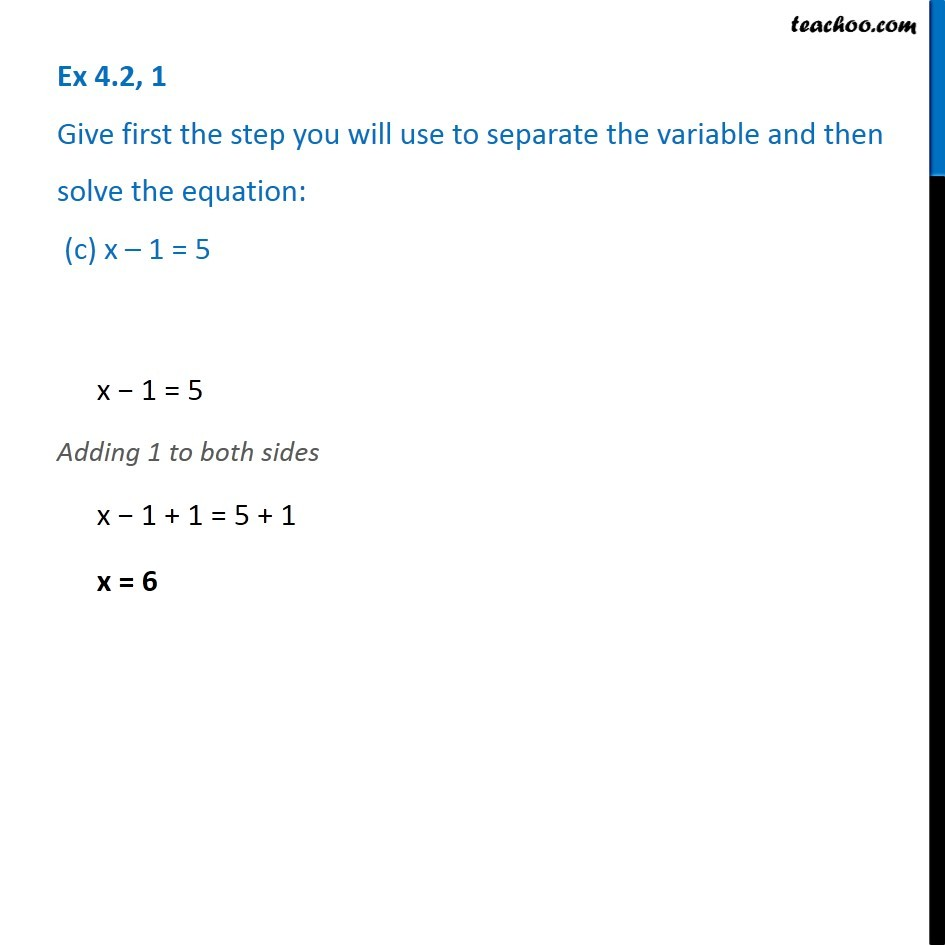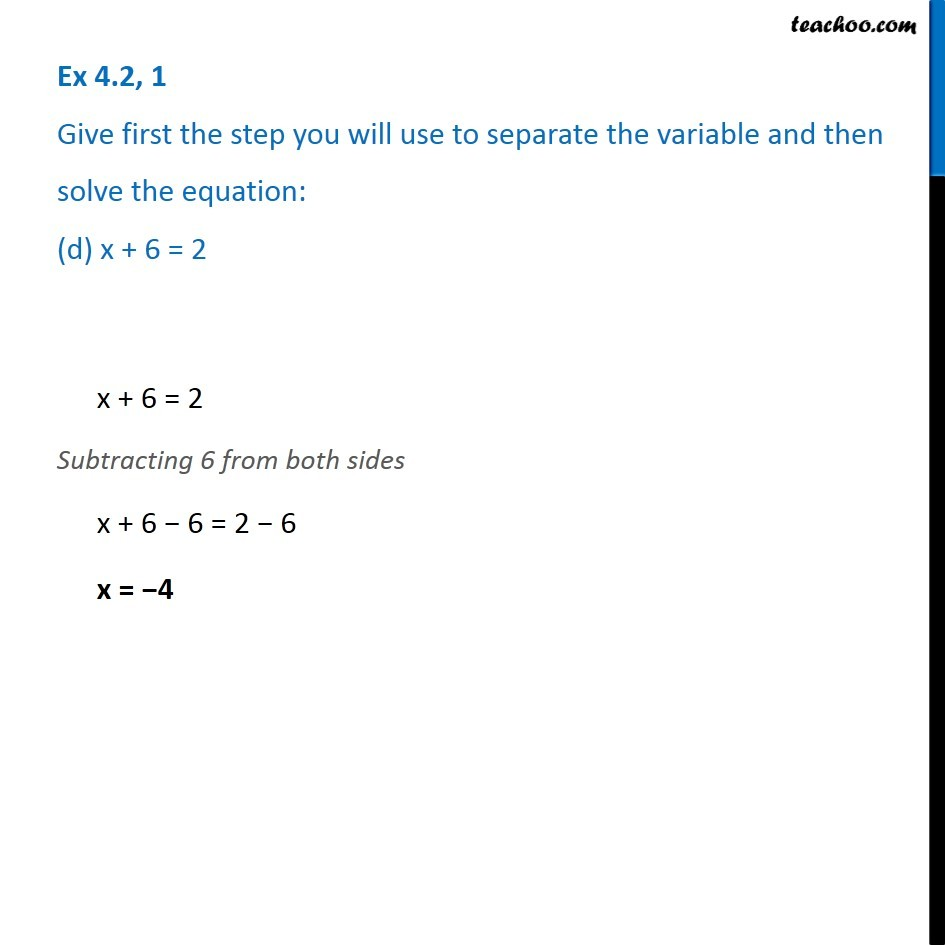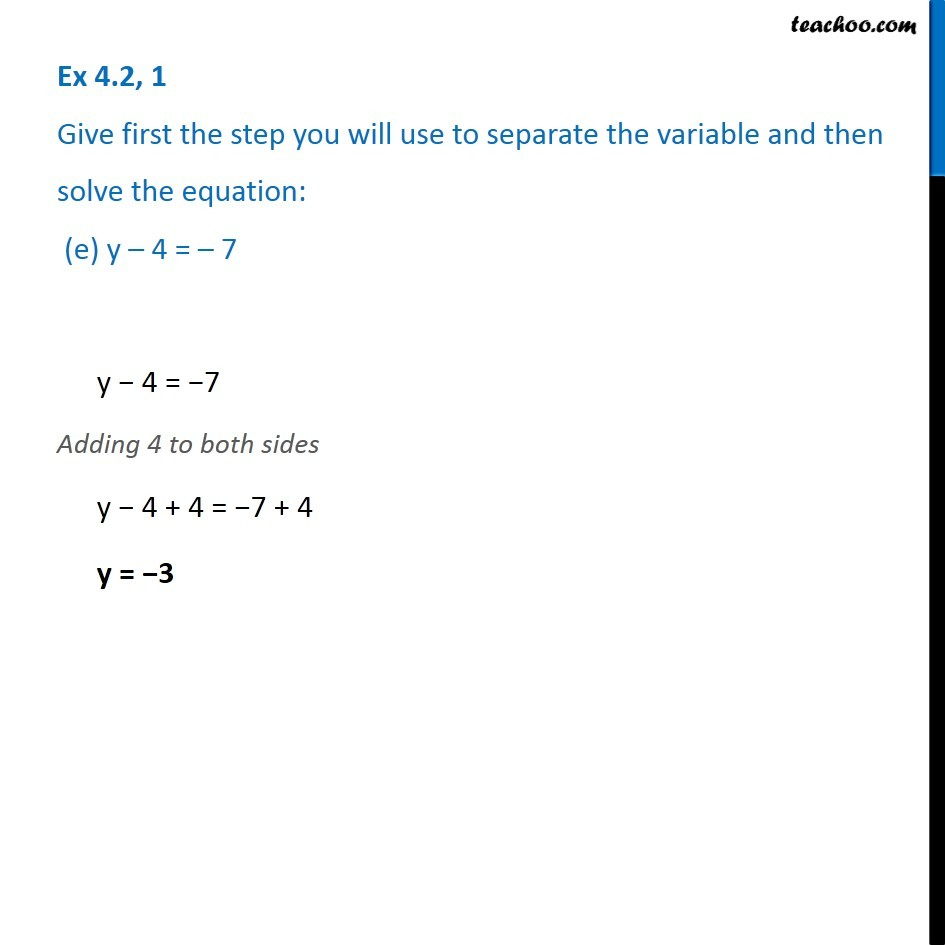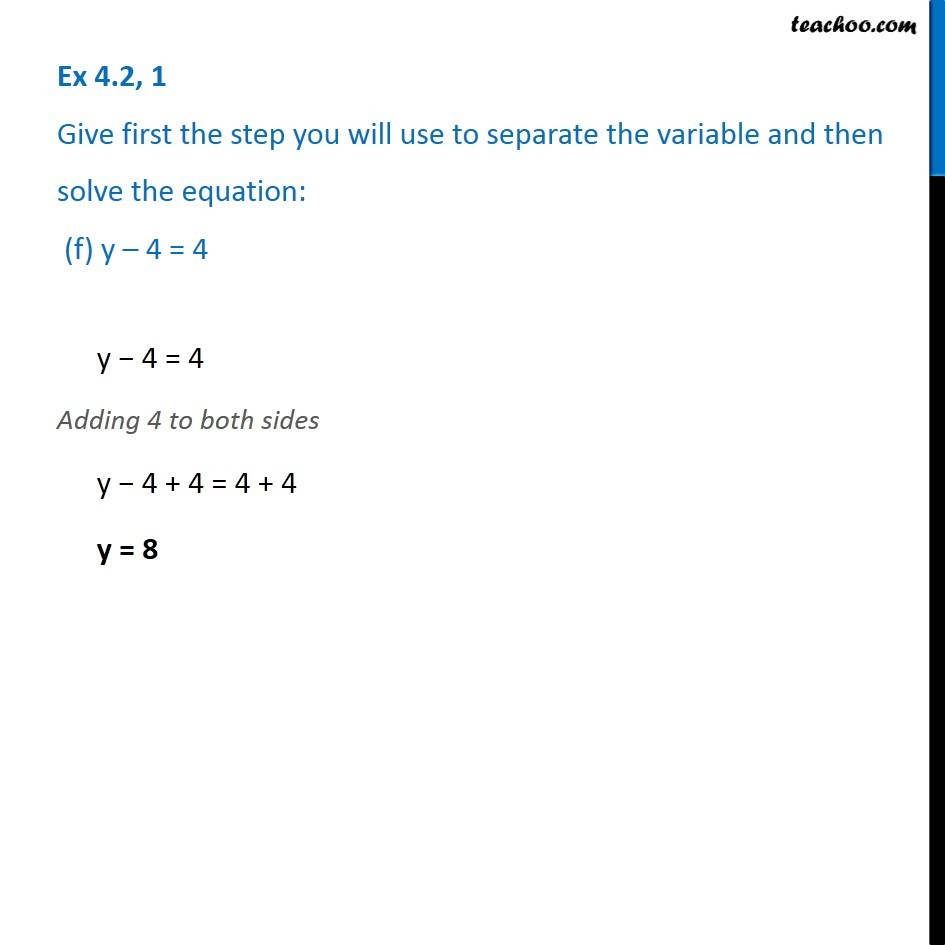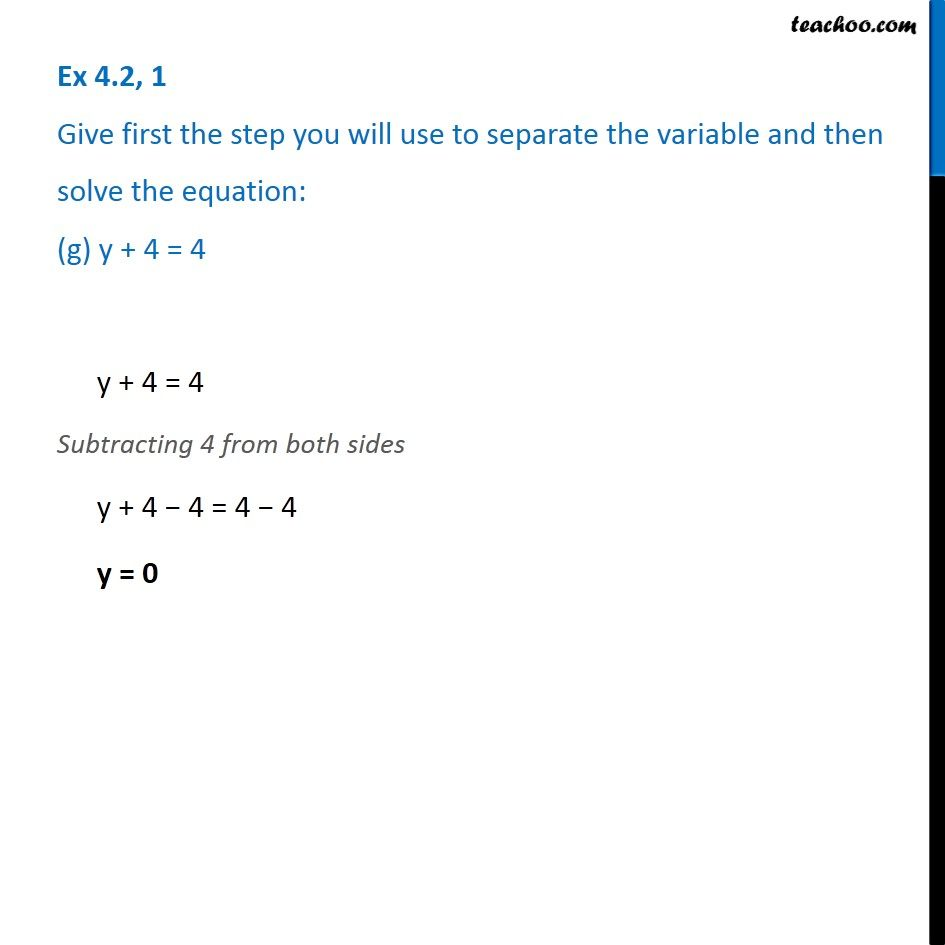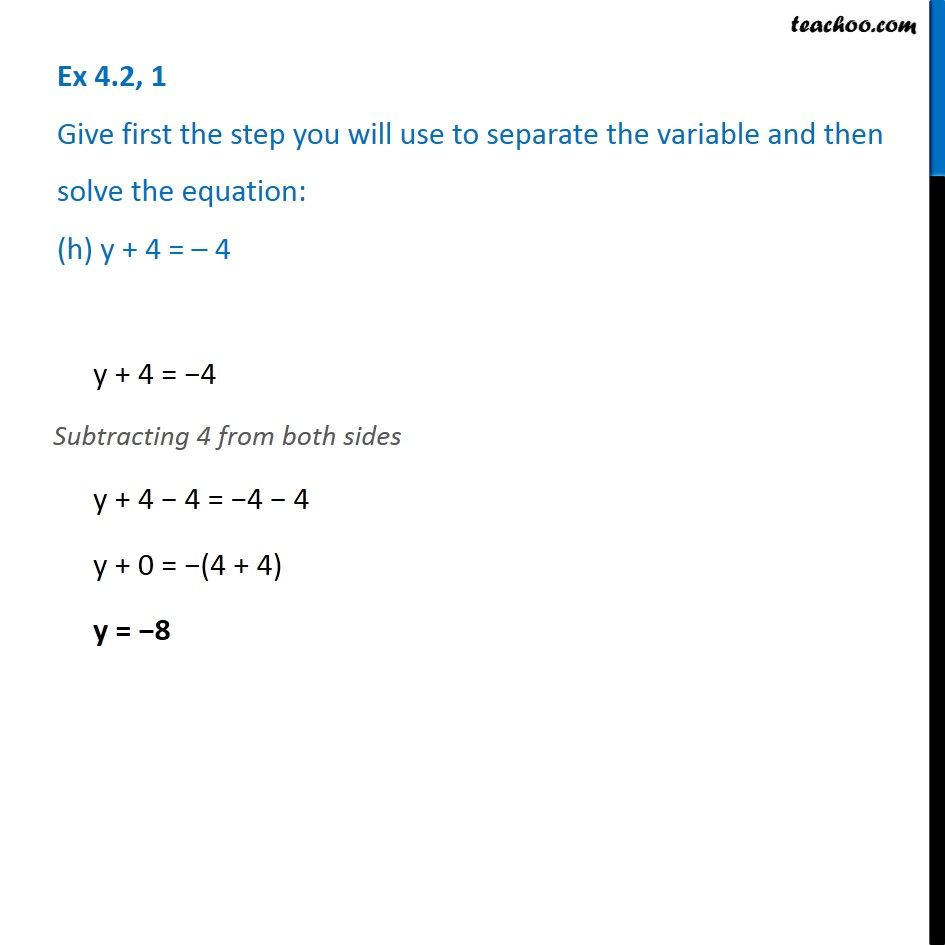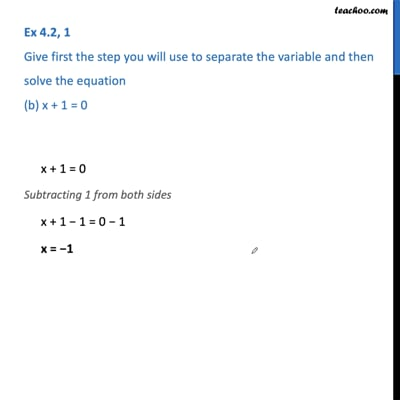This video is only available for Teachoo black users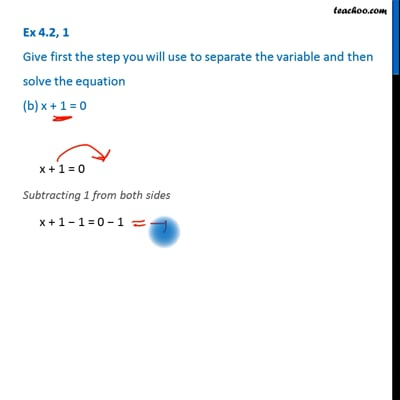This video is only available for Teachoo black users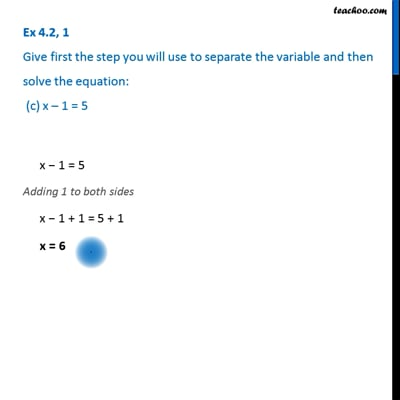This video is only available for Teachoo black users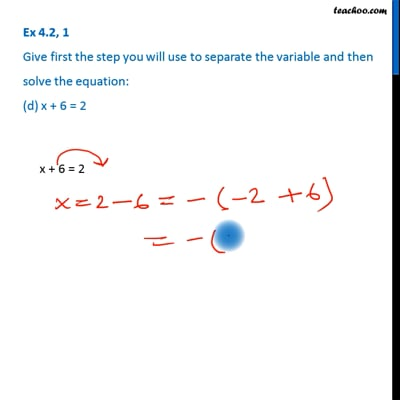This video is only available for Teachoo black usersThis video is only available for Teachoo black users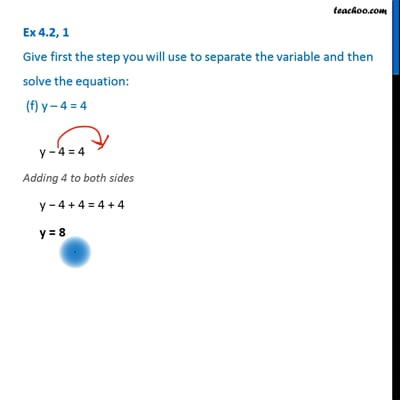This video is only available for Teachoo black users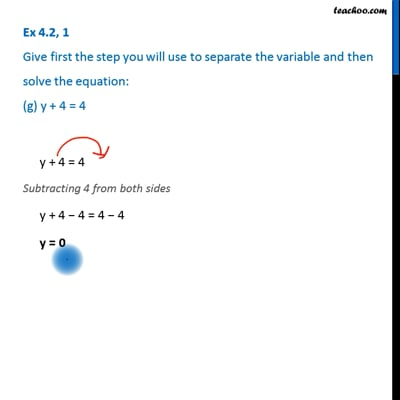This video is only available for Teachoo black users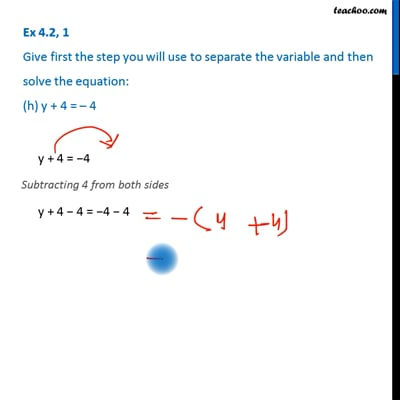This video is only available for Teachoo black users

Learn in your speed, with individual attention - Teachoo Maths 1-on-1 Class

### Transcript

Ex 4.2, 1 Give first the step you will use to separate the variable and then solve the equation: (a) x 1 = 0x 1 = 0 Adding 1 to both sides x 1 + 1 = 0 + 1 x = 1 Ex 4.2, 1 Give first the step you will use to separate the variable and then solve the equation (b) x + 1 = 0x + 1 = 0 Subtracting 1 from both sides x + 1 1 = 0 1 x = 1 Ex 4.2, 1 Give first the step you will use to separate the variable and then solve the equation: (c) x 1 = 5x 1 = 5 Adding 1 to both sides x 1 + 1 = 5 + 1 x = 6 Ex 4.2, 1 Give first the step you will use to separate the variable and then solve the equation: (d) x + 6 = 2x + 6 = 2 Subtracting 6 from both sides x + 6 6 = 2 6 x = 4 Ex 4.2, 1 Give first the step you will use to separate the variable and then solve the equation: (e) y 4 = 7y 4 = 7 Adding 4 to both sides y 4 + 4 = 7 + 4 y = 3 Ex 4.2, 1 Give first the step you will use to separate the variable and then solve the equation: (f) y 4 = 4 y 4 = 4 Adding 4 to both sides y 4 + 4 = 4 + 4 y = 8 Ex 4.2, 1 Give first the step you will use to separate the variable and then solve the equation: (g) y + 4 = 4 y + 4 = 4 Subtracting 4 from both sides y + 4 4 = 4 4 y = 0 Ex 4.2, 1 Give first the step you will use to separate the variable and then solve the equation: (h) y + 4 = 4 y + 4 = 4 Subtracting 4 from both sides y + 4 4 = 4 4 y + 0 = (4 + 4) y = 8